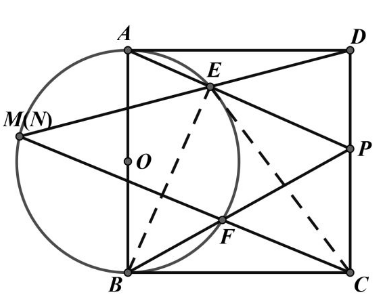【答案】

\begin{aligned} & D E \cdot D M=D A^2=D C^2 \\ & \therefore \triangle D N C \sim \triangle D C E \\ & \therefore \angle D C E=\angle D N C \end{aligned}
$B 、 C 、 P 、 E$ 四点共圆，
\begin{aligned} & \therefore \angle D C E=\angle P B E=\angle F N E \\ & \therefore \angle D N C=\angle F N E \end{aligned}
$\therefore N 、 F 、 C$ 三点共线, 即 $D E 、 C F$ 的交点为
$N, M$ 与 $N$ 重合。